# A Lane Detection Approach for Self-Driving Vehicles

Abstract: Traffic accidents have become one of the most serious problems in today’s world. Increase in the number of vehicles, human errors towards traffic rules and the difficulty to oversee situational dangers by drivers are contributing to the majority of accidents on the road. Lane detection is an essential component for autonomous vehicles. In this paper, I have designed and implemented an automatic lane marking detection algorithm. Various experiments in a U.S. context show that the proposed approach can be very effective in lane detection. The algorithm is verified for both white lanes and yellow lanes by improving color detection and averaging temporal patterns.

### Getting Started

We will develop a simple pipeline using OpenCV and Python for finding lane lines in an image, then apply this pipeline to a full video feed. Udacity’s Self-Driving Car program got me started with these skills and I highly recommend this program if you want to get into this field.

We will be leveraging the popular SciPy and NumPy packages for doing scientific computations and the OpenCV package for computer vision algorithms:

`import matplotlib.pyplot as pltimport numpy as npimport cv2`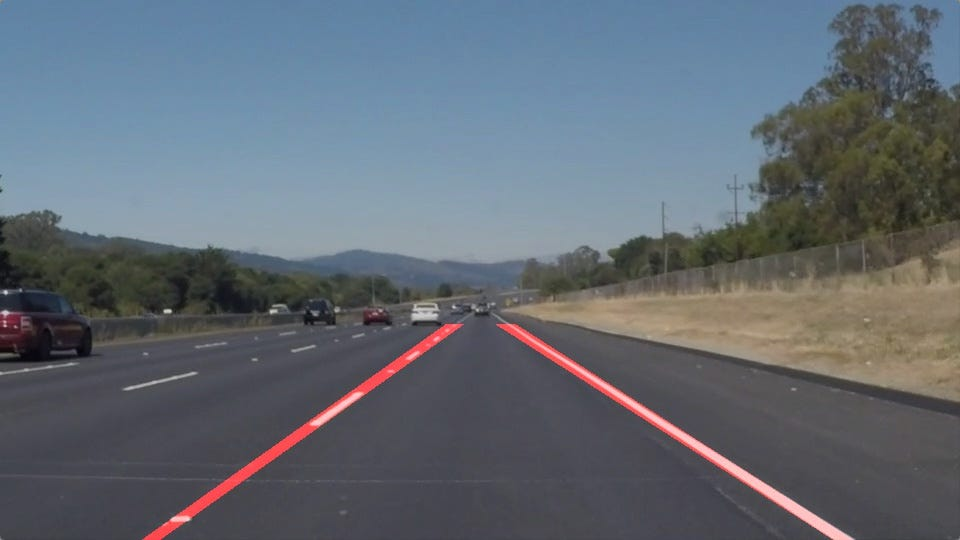Figure 1: an unprocessed frame (left) and a frame with lanes automatically indicated (right).

### Lane Detection Pipeline

The first iteration of the lane detection algorithm works well on non-curved roads with a main camera mounted on the vehicle dashboard. In these videos, the weather conditions are good and it is daytime. We will handle shadows, continuous and discontinuous lanes with different colors.

Remove noise: Starting with a frame from a video taken from a single camera on the dashboard, we clean up the image by applying a Gaussian blur. This step will remove noise and tiny details from the image such as distant objects that are irrelevant for our purpose.

`def remove_noise(image, kernel_size):    return cv2.GaussianBlur(image, (kernel_size, kernel_size), 0)`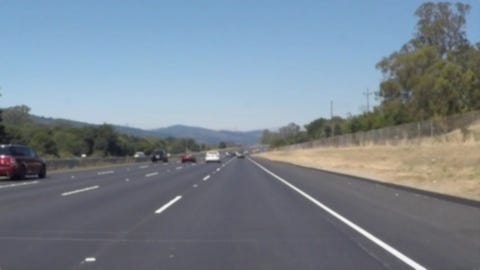Figure. 2: Blur filter applied to image.

Discard color information: The second step is to convert the image to grayscale before isolating the region of interest. This step will highlight pixels with a higher brightness value, including the ones defining marking lanes in the frame.

`def discard_colors(image):    return cv2.cvtColor(image, cv2.COLOR_BGR2GRAY)`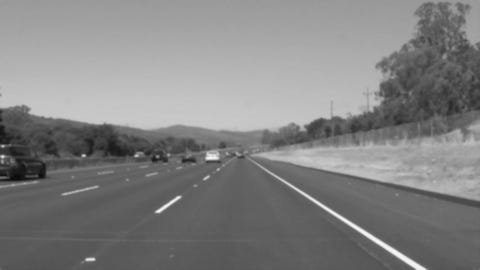Figure. 3: grayscale transformation applied to blurred image.

Detect edges: The next step is to run Canny edge detection. The Canny algorithm detects edges on a picture by looking for quick changes in color between a pixel and its neighbors. The blur and grayscale step will help make the main lane lines stand out. The result will give a black and white image.

`def detect_edges(image, low_threshold, high_threshold):    return cv2.Canny(image, low_threshold, high_threshold)`
`image = detect_edges(gray_image, low_threshold=50, high_threshold=150)plt.imshow(image, cmap='gray')`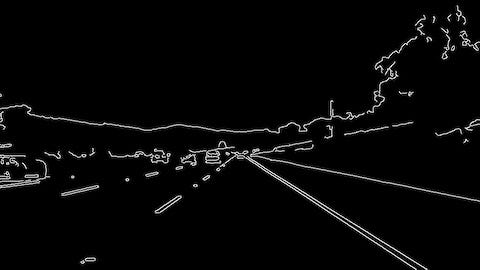Figure. 4: Canny edge detection applied to grayscale image.

Region of interest: It’s important to cut out as much of the noise as possible within a frame. Given the position and orientation of the camera, we can assume that lanes will be located in the lower half of the image. We can just isolate that area using a trapezoid shape. While it may be tempting to hardcode the best possible place to look for lines, lines can move around as you drive. For this reason, a simple ratio to setup a polygonial shape is used. We apply a generous ratio as we don’t want our region to be too narrow resulting in missing lanes out of the region of interest.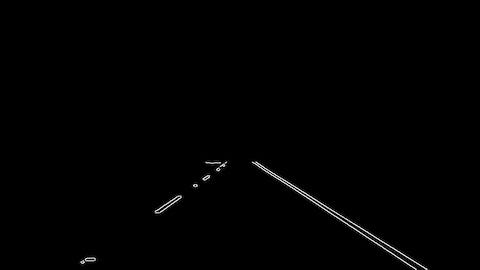Figure. 5: Masking the region of interest.
`def region_of_interest(image, vertices):    # defining a blank mask to start with mask = np.zeros_like(image)    # defining a 3 channel or 1 channel color to fill the mask with depending on the input image`
`    if len(image.shape) > 2:        channel_count = image.shape  # i.e. 3 or 4 depending on your image        ignore_mask_color = (255,) * channel_count    else:        ignore_mask_color = 255`
`    # filling pixels inside the polygon defined by "vertices" with the fill color`
`    cv2.fillPoly(mask, vertices, ignore_mask_color) .     # returning the image only where mask pixels are non-zero`
`    masked_image = cv2.bitwise_and(image, mask)    return masked_image`
`xsize = img.shapeysize = img.shape`
`dx1 = int(0.0725 * xsize)dx2 = int(0.425 * xsize)dy = int(0.6 * ysize)`
`# calculate vertices for region of interest`
`vertices = np.array([[(dx1, ysize), (dx2, dy), (xsize - dx2, dy), (xsize - dx1, ysize)]], dtype=np.int32)`
`image = region_of_interest(image, vertices)`

Draw lines: This function draws lines with color and thickness
Lines are drawn on the image in-place. If you want to make the lines semi-transparent, think about combining this function with the weighted_image() function given further down.

`def draw_lines(image, lines, color=[255, 0, 0], thickness=2):    for line in lines:        for x1,y1,x2,y2 in line:            cv2.line(image, (x1, y1), (x2, y2), color, thickness)`

Hough transform: Using probabilistic Hough lines, we identify the location of lane lines on the road. The Hough transform algorithm extracts all the lines passing through each of our edge points and group them by similarity. The HoughLinesP function in OpenCV returns an array of lines organized by endpoints (x1, x1, x2, x2). It’s important to understand that we are no longer viewing point in the (x, y) cartesian coordinate system but are now viewing points in the (ρ, θ) polar coordinate system that the Hough transform operates in. Understanding the internals of this transform helps manipulating parameters provided to the function.

`def hough_lines(image, rho, theta, threshold, min_line_len, max_line_gap):    lines = cv2.HoughLinesP(image, rho, theta, threshold, np.array([]), minLineLength=min_line_len, maxLineGap=max_line_gap)    return lines`
`rho = 0.8theta = np.pi/180threshold = 25min_line_len = 50max_line_gap = 200`
`lines = hough_lines(img, rho, theta, threshold, min_line_len, max_line_gap)`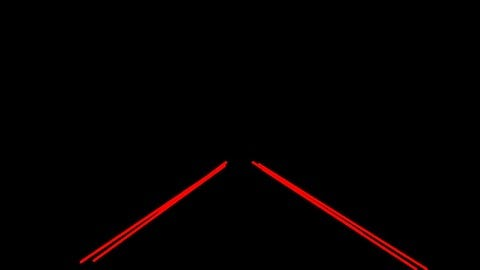Figure. 6: Hough transformation returns a list of lines.

Sort lines: The Hough transformation gives us back multiple lines, but we only want two distinct lanes for our car to drive in between. We have two distinct sets we want to detect: the left and the right lane markers. In this section, we organize lines by slope. The slope of a line is given by:

We use this equation to organize lines by their slope. Positive slopes are for the right lane and negative slopes are for the left lane.

``def slope(x1, y1, x2, y2):    return (y1 - y2) / (x1 - x2)``
`def separate_lines(lines):    right = []    left = []`
`    for x1,y1,x2,y2 in lines[:, 0]:        m = slope(x1,y1,x2,y2)        if m >= 0:            right.append([x1,y1,x2,y2,m])        else:            left.append([x1,y1,x2,y2,m])    return right, left`
`right_lines, left_lines = separate_lines(lines)`

Reject outliers: We then filter out lines with unacceptable slopes that throw off the intended slope of each line.

`def reject_outliers(data, cutoff, threshold=0.08):    data = np.array(data)    data = data[(data[:, 4] >= cutoff) & (data[:, 4] <= cutoff)]    m = np.mean(data[:, 4], axis=0)    return data[(data[:, 4] <= m+threshold) & (data[:, 4] >= m-threshold)]`
`if right_lines and left_lines:    right = reject_outliers(right_lines,  cutoff=(0.45, 0.75))    left = reject_outliers(left_lines, cutoff=(-0.85, -0.6))`

Run linear regression: We finally merge left and right sets using linear regression. Linear regression is an attempt at finding the best relationship between a group of points. We are getting a line that passes at the closest possible distance from each point. We apply linear regression on both the left and right sets of lines.

`def lines_linreg(lines_array):    x = np.reshape(lines_array[:, [0, 2]], (1, len(lines_array) * 2))    y = np.reshape(lines_array[:, [1, 3]], (1, len(lines_array) * 2))    A = np.vstack([x, np.ones(len(x))]).T    m, c = np.linalg.lstsq(A, y)    x = np.array(x)    y = np.array(x * m + c)    return x, y, m, c`
`x, y, m, c = lines_linreg(lines)`
`# This variable represents the top-most point in the image where we can reasonable draw a line to.`
`min_y = np.min(y)`
`# Calculate the top point using the slopes and intercepts we got from linear regression.`
`top_point = np.array([(min_y - c) / m, min_y], dtype=int)`
`# Repeat this process to find the bottom left point.`
`max_y = np.max(y)bot_point = np.array([(max_y - c) / m, max_y], dtype=int)`

Span lines: Using some simple geometry (y = mx + b), we calculate extrema. We use the result of the linear regression to extrapolate to those extrema. We extend the left and right lines off across the image and clip the line using our previous region of interest.

`def extend_point(x1, y1, x2, y2, length):    line_len = np.sqrt((x1 - x2)**2 + (y1 - y2)**2)    x = x2 + (x2 - x1) / line_len * length    y = y2 + (y2 - y1) / line_len * length    return x, y`
`x1e, y1e = extend_point(bot_point,bot_point,top_point,top_point, -1000) # bottom point`
`x2e, y2e = extend_point(bot_point,bot_point,top_point,top_point,  1000) # top point`
`# return the line.`
`line = np.array([[x1e,y1e,x2e,y2e]])return np.array([line], dtype=np.int32)`

Draw the lines and return the final image: The final step is to superimpose the left and right lines onto the original image to visually validate the correctness and accuracy of our pipeline implementation using a weighted computation for each pixel.The result image is computed as follows:

initial_image * α + image * β + γ

NOTE: initial_image and image must be the same shape!

`def weighted_image(image, initial_image, α=0.8, β=1., λ=0.):    return cv2.addWeighted(initial_image, α, image, β, λ)`
`line_image = np.copy((image)*0)draw_lines(line_image, lines, thickness=3)line_image = region_of_interest(line_image, vertices)final_image = weighted_image(line_image, image)return final_image`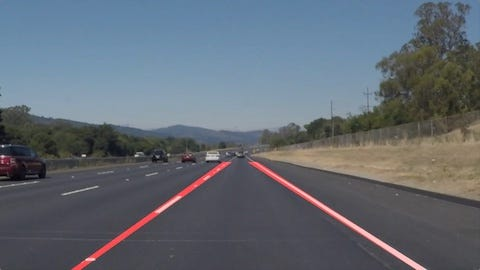Figure. 6: Masking the region of interest.

### Advanced Techniques

#### Color Transform

In the above version of the pipeline, we decided to discard color information and rely exclusively on pixel brightness to detect lane marking on the road. It works well during daylight and with a simple terrain but we observed that the lane detection accuracy drops significantly in less ideal conditions. By transforming the original image from RGB colorspace to HSV colorspace (more info about HSL and HSV here), we want to filter out pixels which are not the given color of the lanes. Using hue and saturation values, not how dark a pixel is, will ensure lines of a given color are more easily detected in shaded or low-contrast regions.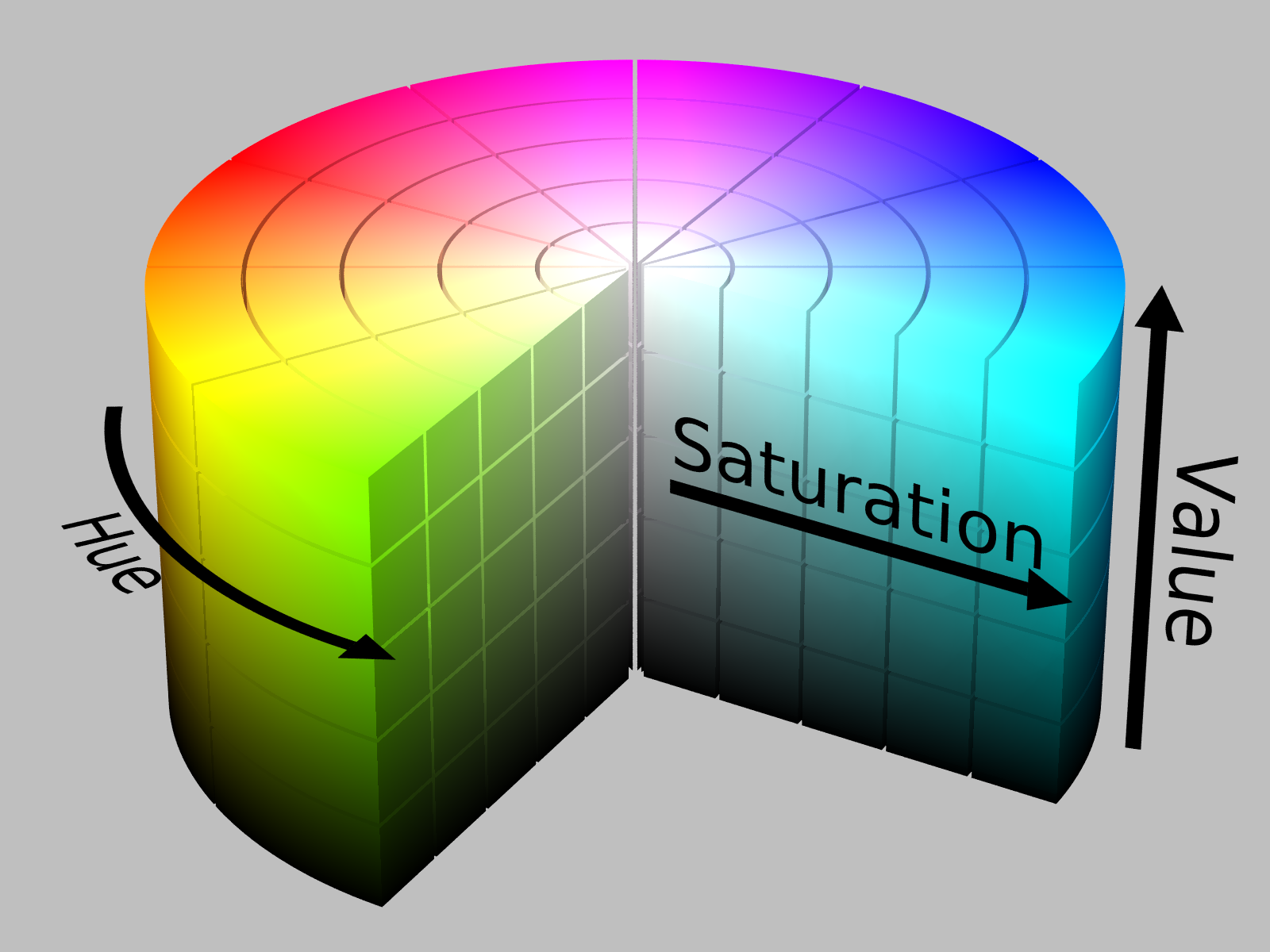Figure 7: HSV colorspace representation.

We create two color filters that will extract the whites and yellows in the image and apply them to turn black any other pixels.

`def filter_image(image):    hsv_image = cv2.cvtColor(image,cv2.COLOR_BGR2HSV)`
`    sensitivity = 51    lower_white = np.array([0,0,255-sensitivity])    upper_white = np.array([255,sensitivity,255])`
`    lower_yellow = np.array([18,102,204], np.uint8)    upper_yellow = np.array([25,255,255], np.uint8)`
`    white_mask = cv2.inRange(hsv_image, lower_white, upper_white)    yellow_mask = cv2.inRange(hsv_image, lower_yellow, upper_yellow)`
`    filtered_image = cv2.bitwise_and(image, image, mask=white_mask+yellow_mask)    return filtered_image`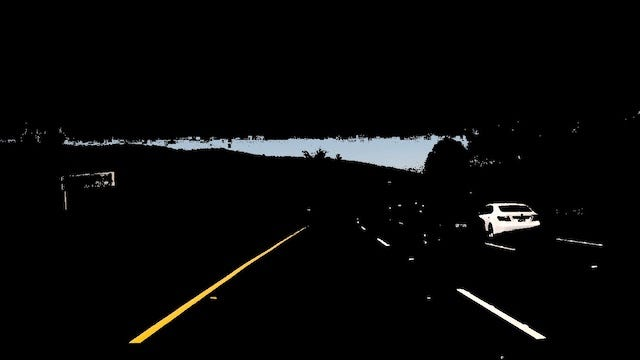Figure 8: RGB colorspace to HSV colorspace.

#### Previous Frame Averaging

On the video footage, we will average the previous frame lines with the current frame lines to improve our lane detection algorithm. Remembering each last frame lines will prevent jittery using average and provide a solution when our pipeline fails to detect lines in less than ideal conditions.

`global prev_leftglobal prev_right`
`prev_left = Noneprev_right = None`
`def pipeline(image):    global prev_left    global prev_right`
`right = reject_outliers(right_lines,  cutoff=(0.45, 0.75))`
`if len(right) != 0:    right = merge_lines(right)    right = merge_prev(right, prev_right)    prev_right = rightelse:    right = prev_right`
`left = reject_outliers(left_lines, cutoff=(-0.85, -0.6))`
`if len(left) != 0:    left = merge_lines(left)    left = merge_prev(left, prev_left)    prev_left = leftelse:    left = prev_left`

### Final video outputs

The pipeline above works well on non-curved roads with the camera mounted on the vehicle dashboard in a stationary position. More work will need to be done to make this work on curved roads, under different lighting and weather conditions and with a camera mounted at different points.

### Conclusion

In this project, I have developed and implemented an algorithm for detecting white and yellow colored lanes on the road. The lane detection method is robust and effective in finding the exact lanes by using both color and edge orientations. The main contributions are the color segmentation procedure identifying the yellow or white colored lanes followed by edge orientation in which the boundaries are eliminated, lanes are detected, left and right regions are labeled, outliers are removed and finally one line per region remains after using a linear regression on each set. As the camera remains constant with respect to the road surface, the road portion of the image can be exclusively cropped by providing coordinates, so that identifying the lanes becomes much more efficient. The experimental results show the effectiveness of the proposed method in cases of yellow and white colored lanes. The entire work is done in a static way using static images and extended to detect lanes in videos.

The code repository for this project can be found here. Our next project will be about “traffic sign classification”: how to train a convolutional neural network to classify traffic signs. See you there.